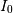numpy.i0¶

numpy.i0(x)[source]

Modified Bessel function of the first kind, order 0.

Usually denoted. This function does broadcast, but will not “up-cast” int dtype arguments unless accompanied by at least one float or complex dtype argument (see Raises below).

Parameters : x : array_like, dtype float or complex Argument of the Bessel function. out : ndarray, shape = x.shape, dtype = x.dtype The modified Bessel function evaluated at each of the elements of x. TypeError: array cannot be safely cast to required type If argument consists exclusively of int dtypes.

scipy.special.iv, scipy.special.ive

Notes

We use the algorithm published by Clenshaw [R29] and referenced by Abramowitz and Stegun [R30], for which the function domain is partitioned into the two intervals [0,8] and (8,inf), and Chebyshev polynomial expansions are employed in each interval. Relative error on the domain [0,30] using IEEE arithmetic is documented [R31] as having a peak of 5.8e-16 with an rms of 1.4e-16 (n = 30000).

References

 [R29] (1, 2) C. W. Clenshaw, “Chebyshev series for mathematical functions”, in National Physical Laboratory Mathematical Tables, vol. 5, London: Her Majesty’s Stationery Office, 1962.
 [R30] (1, 2) M. Abramowitz and I. A. Stegun, Handbook of Mathematical Functions, 10th printing, New York: Dover, 1964, pp. 379. http://www.math.sfu.ca/~cbm/aands/page_379.htm

Examples

>>> np.i0([0.])
array(1.0)
>>> np.i0([0., 1. + 2j])
array([ 1.00000000+0.j        ,  0.18785373+0.64616944j])